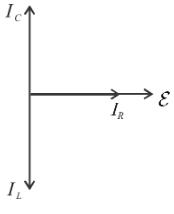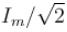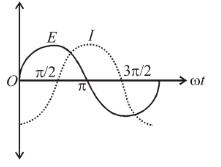Courses

# Alternating Current MCQ Level - 1

## 10 Questions MCQ Test Topic wise Tests for IIT JAM Physics | Alternating Current MCQ Level - 1

Description
This mock test of Alternating Current MCQ Level - 1 for Physics helps you for every Physics entrance exam. This contains 10 Multiple Choice Questions for Physics Alternating Current MCQ Level - 1 (mcq) to study with solutions a complete question bank. The solved questions answers in this Alternating Current MCQ Level - 1 quiz give you a good mix of easy questions and tough questions. Physics students definitely take this Alternating Current MCQ Level - 1 exercise for a better result in the exam. You can find other Alternating Current MCQ Level - 1 extra questions, long questions & short questions for Physics on EduRev as well by searching above.
QUESTION: 1

Solution:

QUESTION: 2

### An alternating emf is applied across a parallel combination of a resistance R, capacitance C and an induction L. If IR, IL, IC are the currents through R, L and C respectively, then the diagram which correctly represents the phase relationship among IR, IL, IC and source emf ε is the given by

Solution:

IL lags behind IR by a phase of π/2
IC lags behind IR by a phase of π/2QUESTION: 3

### The average power dissipated is pure inductance is

Solution:
QUESTION: 4

The value of current at half power points is

Solution:

The correct answer is:QUESTION: 5

A DC ammeter cannot measure alternating current value

Solution:
QUESTION: 6

The time required for a 50Hz alternating current to increase from zero to 70.7% of its peak value is

Solution:

Solution :- 1/(50×8)

= 1/400sec

= 2.5ms

QUESTION: 7

The variation of the instantaneous current I and the instantaneous emf E in a circuit is as shown in figure. Which of the following statements is correctSolution:

QUESTION: 8

The term cos φ in an AC circuit is called

Solution:

The correct answer is: power factor

QUESTION: 9

In a series LCR circuit containing an AC voltage source of frequency ω, current and voltage V. Then for the given resistance, the correct statement is:

Solution:

The correct answer is: Current is maximum at ω2 = 1/LC

QUESTION: 10

In an electric circuit applied ac emf is ε = 20 sin 300t Volt and the current is i = 4 sin 300t Amp. The resistance of the circuit is.

Solution: